This mathematics ClipArt gallery offers 19 illustrations of images used in trigonometry. Included are images of projectiles, vectors, and arc length.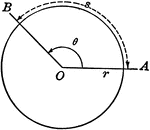### Model of Arc Length, Angle Measure, and Radius

Illustration showing that the arc length can be found by multiplying the angle measure by the radius…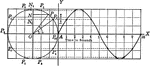### Projection of Points in Circular Motion

Illustration of the projection of point P as it moves around a vertical circle of radius 3 in. in a…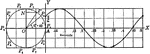### Projection of Points in Circular Motion

Illustration of the projection of point P as it moves around a vertical circle of radius 2 ft. in a…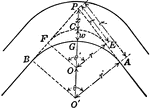### Curve in Pavement of Road

Illustration of blueprint used by highway engineers to widen the pavement on the inside of the curve…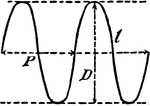### Trigonometric Curve

A curve that is a function and resembles the sine or cosine curve.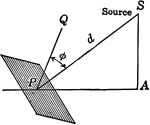### Illumination of a Surface When the Surface is not Perpendicular to the Source

Illustration modeling the illumination on a surface when the surface is not perpendicular to hte rays…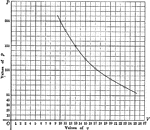### Logarithmic Graph of the Law of Expansion

Logarithmic graph (smooth curve) modeling the law of expansion with values of volume and pressure.### Path of Projectile

Illustration modeling the path of a projectile.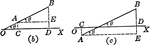### Projection of a Line Segment

CD is the projection of AB upon OX. In each case AE=CD and AE=ABcos theta.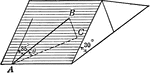### Roof With 30 degree Inclination for Trigonometry Triangle Problems

Roof with a slope of 30 degrees. Angle theta is the inclination to the horizontal of the line AB, drawn…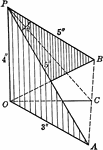### Two Squares With Sides of Lengths 3,4,5 Placed at Right Angles to Each Other

Two set squares, whose sides are 3,4, and 5 in., are placed so that their 4-in. sides and right angles…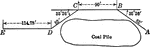### Survey of Lake Front in Chicago

Illustration showing a survey on the lake front in Chicago with distances and angle measures shown.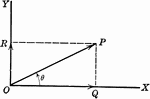### Vector

OP is a line representing a vector (directed quantity)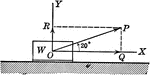### Vector Showing Force at Angle of 20 degrees

OP is a vector (directed quantity) showing the force by a weight acting on it at an angle of 20 degrees.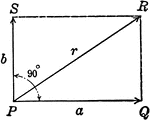### Resultant Vector With Vectors at 90 degrees

Illustration of the resultant vector when two vectors are acting upon a body at point P at 90 degrees.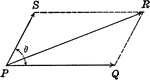### Resultant Vectors

Illustration of the resultant vector when two vectors are acting upon a body at point P.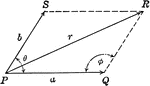### Resultant Vector With Vectors at Angle Ǝ

Illustration of the resultant vector when two vectors are acting upon a body at point P at Ǝ degrees.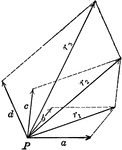### Resultant Vector From 4 Forces

Illustration of the resultant vector when four vectors are acting upon a body at point P.